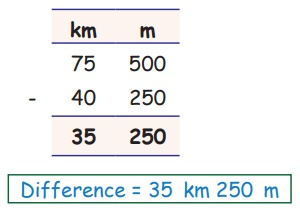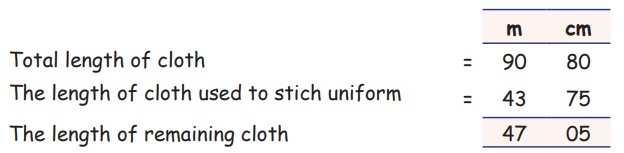Home | | Maths 5th Std | Subtraction of length

# Subtraction of length

5th Maths : Term 1 Unit 4 : Measurements : Subtraction of length

Subtraction

Examples

Find the difference

i) 75 km 500m − 40 km 250 mDifference = 35 km 250 m

ii) 55 km 75 cm − 23m 40cmDiffernce = 32 m 35cm

Try this

Subtract the following

1. 1075 km 400m −27 km 350 m = 1048 km 50m

2. 250 m 25cm −127m 18cm = 123 m 07 cm

3. 27 km 900m − 18km 850 m = 9km 050 m

Example

Kannan bought 90 m 80 cm of cloth .He used to stich uniform of 43 m 75 cm. How much cloth is left?

Solution:The length of the remaining cloth is 47 m 05 cm.

Tags : Measurements | Term 1 Chapter 4 | 5th Maths , 5th Maths : Term 1 Unit 4 : Measurements
Study Material, Lecturing Notes, Assignment, Reference, Wiki description explanation, brief detail
5th Maths : Term 1 Unit 4 : Measurements : Subtraction of length | Measurements | Term 1 Chapter 4 | 5th Maths